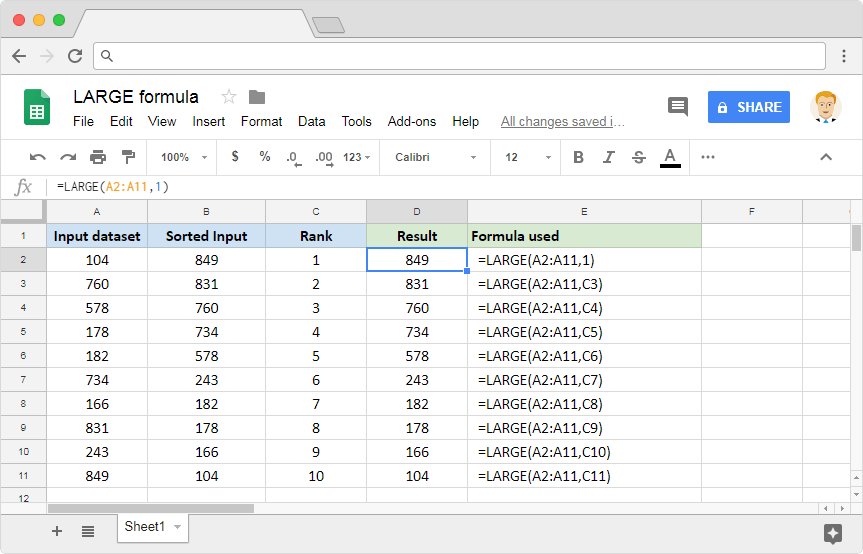# How to use the LARGE formula in Google SheetsNumber crunching is one of the primary activities that the spreadsheets applications are built for. We all know how to find out the maximum value from a dataset, using the MAX formula. What if we need to find out the second largest value from the set of numeric values? Or, the third, or nth largest value? Is there any readymade tool available? Thankfully, there is. The LARGE formula in Google Sheets is the exact tool that we need here.

### Syntax

LARGE(data, n)

• data – is the reference to the range or cells that contain the numeric dataset.
• n – is the rank from largest to smallest of the element that the formula has to return. For instance, if we need the 3rd largest value from the dataset, we should set this parameter to 3.

If we need the largest value, set n to 1. On the other hand, if we need the smallest value from a set of 15 numbers, then set n to 15.

### Usage: LARGE formula in Google Sheets

On a quick look, the syntax looks like it isn’t that difficult to apply and understand. Nevertheless, let’s go and try our hands on the Google Sheets application. Please take a look at the following snapshot.While the first parameter accepts only range references, the second parameter can take both direct numbers as well as cell references.

We have the input dataset laid out on column A, which will serve as the source for the first parameter. We have placed the same dataset on column B, except we sorted it in the descending order. Column B is just for reference so that we can quickly compare the outputs with the expected output.

Now let us quickly go through the column D. On the cell D2, we are looking to extract the first largest value. On D3, the second largest value and so on and so forth. If we were to compare columns B and D, we will notice that the LARGE formula returns the outputs the exact manner that it is expected to do so.